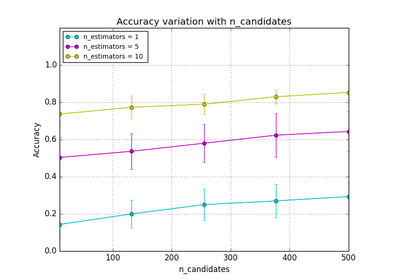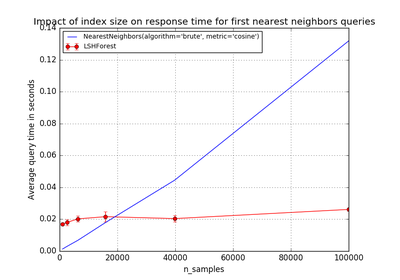# `sklearn.neighbors`.LSHForest¶

class `sklearn.neighbors.``LSHForest`(n_estimators=10, radius=1.0, n_candidates=50, n_neighbors=5, min_hash_match=4, radius_cutoff_ratio=0.9, random_state=None)[source]

Performs approximate nearest neighbor search using LSH forest.

LSH Forest: Locality Sensitive Hashing forest  is an alternative method for vanilla approximate nearest neighbor search methods. LSH forest data structure has been implemented using sorted arrays and binary search and 32 bit fixed-length hashes. Random projection is used as the hash family which approximates cosine distance.

The cosine distance is defined as `1 - cosine_similarity`: the lowest value is 0 (identical point) but it is bounded above by 2 for the farthest points. Its value does not depend on the norm of the vector points but only on their relative angles.

Read more in the User Guide.

Parameters: n_estimators : int (default = 10) Number of trees in the LSH Forest. min_hash_match : int (default = 4) lowest hash length to be searched when candidate selection is performed for nearest neighbors. n_candidates : int (default = 10) Minimum number of candidates evaluated per estimator, assuming enough items meet the min_hash_match constraint. n_neighbors : int (default = 5) Number of neighbors to be returned from query function when it is not provided to the `kneighbors` method. radius : float, optinal (default = 1.0) Radius from the data point to its neighbors. This is the parameter space to use by default for the :meth`radius_neighbors` queries. radius_cutoff_ratio : float, optional (default = 0.9) A value ranges from 0 to 1. Radius neighbors will be searched until the ratio between total neighbors within the radius and the total candidates becomes less than this value unless it is terminated by hash length reaching min_hash_match. random_state : int, RandomState instance or None, optional (default=None) If int, random_state is the seed used by the random number generator; If RandomState instance, random_state is the random number generator; If None, the random number generator is the RandomState instance used by np.random. hash_functions_ : list of GaussianRandomProjectionHash objects Hash function g(p,x) for a tree is an array of 32 randomly generated float arrays with the same dimension as the data set. This array is stored in GaussianRandomProjectionHash object and can be obtained from `components_` attribute. trees_ : array, shape (n_estimators, n_samples) Each tree (corresponding to a hash function) contains an array of sorted hashed values. The array representation may change in future versions. original_indices_ : array, shape (n_estimators, n_samples) Original indices of sorted hashed values in the fitted index.

References

 [R237] M. Bawa, T. Condie and P. Ganesan, “LSH Forest: Self-Tuning Indexes for Similarity Search”, WWW ‘05 Proceedings of the 14th international conference on World Wide Web, 651-660, 2005.

Examples

```>>> from sklearn.neighbors import LSHForest
```
```>>> X_train = [[5, 5, 2], [21, 5, 5], [1, 1, 1], [8, 9, 1], [6, 10, 2]]
>>> X_test = [[9, 1, 6], [3, 1, 10], [7, 10, 3]]
>>> lshf = LSHForest(random_state=42)
>>> lshf.fit(X_train)
LSHForest(min_hash_match=4, n_candidates=50, n_estimators=10,
random_state=42)
>>> distances, indices = lshf.kneighbors(X_test, n_neighbors=2)
>>> distances
array([[ 0.069...,  0.149...],
[ 0.229...,  0.481...],
[ 0.004...,  0.014...]])
>>> indices
array([[1, 2],
[2, 0],
[4, 0]])
```

Methods

 `fit`(X[, y]) Fit the LSH forest on the data. `get_params`([deep]) Get parameters for this estimator. `kneighbors`(X[, n_neighbors, return_distance]) Returns n_neighbors of approximate nearest neighbors. `kneighbors_graph`([X, n_neighbors, mode]) Computes the (weighted) graph of k-Neighbors for points in X `partial_fit`(X[, y]) Inserts new data into the already fitted LSH Forest. `radius_neighbors`(X[, radius, return_distance]) Finds the neighbors within a given radius of a point or points. `radius_neighbors_graph`([X, radius, mode]) Computes the (weighted) graph of Neighbors for points in X `set_params`(\*\*params) Set the parameters of this estimator.
`__init__`(n_estimators=10, radius=1.0, n_candidates=50, n_neighbors=5, min_hash_match=4, radius_cutoff_ratio=0.9, random_state=None)[source]
`fit`(X, y=None)[source]

Fit the LSH forest on the data.

This creates binary hashes of input data points by getting the dot product of input points and hash_function then transforming the projection into a binary string array based on the sign (positive/negative) of the projection. A sorted array of binary hashes is created.

Parameters: X : array_like or sparse (CSR) matrix, shape (n_samples, n_features) List of n_features-dimensional data points. Each row corresponds to a single data point. self : object Returns self.
`get_params`(deep=True)[source]

Get parameters for this estimator.

Parameters: deep : boolean, optional If True, will return the parameters for this estimator and contained subobjects that are estimators. params : mapping of string to any Parameter names mapped to their values.
`kneighbors`(X, n_neighbors=None, return_distance=True)[source]

Returns n_neighbors of approximate nearest neighbors.

Parameters: X : array_like or sparse (CSR) matrix, shape (n_samples, n_features) List of n_features-dimensional data points. Each row corresponds to a single query. n_neighbors : int, opitonal (default = None) Number of neighbors required. If not provided, this will return the number specified at the initialization. return_distance : boolean, optional (default = True) Returns the distances of neighbors if set to True. dist : array, shape (n_samples, n_neighbors) Array representing the cosine distances to each point, only present if return_distance=True. ind : array, shape (n_samples, n_neighbors) Indices of the approximate nearest points in the population matrix.
`kneighbors_graph`(X=None, n_neighbors=None, mode='connectivity')[source]

Computes the (weighted) graph of k-Neighbors for points in X

Parameters: X : array-like, shape (n_query, n_features), or (n_query, n_indexed) if metric == ‘precomputed’ The query point or points. If not provided, neighbors of each indexed point are returned. In this case, the query point is not considered its own neighbor. n_neighbors : int Number of neighbors for each sample. (default is value passed to the constructor). mode : {‘connectivity’, ‘distance’}, optional Type of returned matrix: ‘connectivity’ will return the connectivity matrix with ones and zeros, in ‘distance’ the edges are Euclidean distance between points. A : sparse matrix in CSR format, shape = [n_samples, n_samples_fit] n_samples_fit is the number of samples in the fitted data A[i, j] is assigned the weight of edge that connects i to j.

Examples

```>>> X = [, , ]
>>> from sklearn.neighbors import NearestNeighbors
>>> neigh = NearestNeighbors(n_neighbors=2)
>>> neigh.fit(X)
NearestNeighbors(algorithm='auto', leaf_size=30, ...)
>>> A = neigh.kneighbors_graph(X)
>>> A.toarray()
array([[ 1.,  0.,  1.],
[ 0.,  1.,  1.],
[ 1.,  0.,  1.]])
```
`partial_fit`(X, y=None)[source]

Inserts new data into the already fitted LSH Forest. Cost is proportional to new total size, so additions should be batched.

Parameters: X : array_like or sparse (CSR) matrix, shape (n_samples, n_features) New data point to be inserted into the LSH Forest.
`radius_neighbors`(X, radius=None, return_distance=True)[source]

Finds the neighbors within a given radius of a point or points.

Return the indices and distances of some points from the dataset lying in a ball with size `radius` around the points of the query array. Points lying on the boundary are included in the results.

The result points are not necessarily sorted by distance to their query point.

LSH Forest being an approximate method, some true neighbors from the indexed dataset might be missing from the results.

Parameters: X : array_like or sparse (CSR) matrix, shape (n_samples, n_features) List of n_features-dimensional data points. Each row corresponds to a single query. radius : float Limiting distance of neighbors to return. (default is the value passed to the constructor). return_distance : boolean, optional (default = False) Returns the distances of neighbors if set to True. dist : array, shape (n_samples,) of arrays Each element is an array representing the cosine distances to some points found within `radius` of the respective query. Only present if `return_distance=True`. ind : array, shape (n_samples,) of arrays Each element is an array of indices for neighbors within `radius` of the respective query.
`radius_neighbors_graph`(X=None, radius=None, mode='connectivity')[source]

Computes the (weighted) graph of Neighbors for points in X

Neighborhoods are restricted the points at a distance lower than radius.

Parameters: X : array-like, shape = [n_samples, n_features], optional The query point or points. If not provided, neighbors of each indexed point are returned. In this case, the query point is not considered its own neighbor. radius : float Radius of neighborhoods. (default is the value passed to the constructor). mode : {‘connectivity’, ‘distance’}, optional Type of returned matrix: ‘connectivity’ will return the connectivity matrix with ones and zeros, in ‘distance’ the edges are Euclidean distance between points. A : sparse matrix in CSR format, shape = [n_samples, n_samples] A[i, j] is assigned the weight of edge that connects i to j.

Examples

```>>> X = [, , ]
>>> from sklearn.neighbors import NearestNeighbors
>>> neigh.fit(X)
NearestNeighbors(algorithm='auto', leaf_size=30, ...)
>>> A.toarray()
array([[ 1.,  0.,  1.],
[ 0.,  1.,  0.],
[ 1.,  0.,  1.]])
```
`set_params`(**params)[source]

Set the parameters of this estimator.

The method works on simple estimators as well as on nested objects (such as pipelines). The latter have parameters of the form `<component>__<parameter>` so that it’s possible to update each component of a nested object.

Returns: self :

## Examples using `sklearn.neighbors.LSHForest`¶Hyper-parameters of Approximate Nearest NeighborsScalability of Approximate Nearest Neighbors